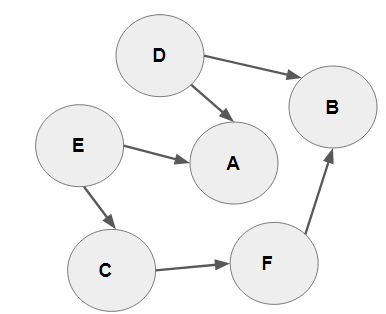# A practical understanding of topological sorting and ordering

The shortest path problem is pivotal in graph theory. It aims at discovering the “most efficient’ or ‘best’ way of moving from x to y, where x and y are both nodes in a given graph. The most efficient or best in this context is evaluated by the lowest sum of edge weights between the path of both vertices. The shortest or quickest path is arrived at by summing the lengths of the individual edges. A best-case scenario is a graph with edges having positive weights. There is also the concept of single-source shortest path problem with s as the source node. For clarity, the source node initiates the transversal within the graph.

There are different algorithms designed to address the shortest path problem. We’ve covered one of such in the Dijkstra algorithm. Topological sorting is an important shortest path algorithm that can be applied to projects that require the completion of a prior task before a new one can be commenced. With this algorithm, there are a series of nodes in a Directed Acyclic Graph (DAG). A directed acyclic graph is a directed graph that contains no cycles. The ordering of nodes using this algorithm is referred to as topological ordering. It is a non-distinct arrangement of nodes that stipulates an edge from x to y should have x appearing before y in the topological sort order.

The source node is quite important with this algorithm and it is usually one with an in-degree of 0 or no incoming edges. Topological sort also works best when a graph consists of positive weights.

Topological sorting on a simple directed acyclic graph

As we’ve explained above, a DAG (Directed Acyclic Graph) contains no cycle or loop. From the graph below, it is quite clear that the edge connections end at vertex A. A topological ordering should naturally commence from nodes D or E. this is because both nodes do not contain an incoming edge.There can be two topological sortings with the above graph. The sorting would always be initiated from the two source nodes of E and D. Are you wondering why the sort should commence with nodes D or E? It is because both nodes do not have an in-degree or incoming edges. With that in mind, in no particular order, the first sort is D, E, C, F, B, A and the second is E, D, C, B, A in the above graph.

The practical application and theories of topological sorting

A practical application of topological sorting is on tasks that involve a sequence of jobs.In a nutshell, when dependencies are required before the completion of a given job, topological sorting may be required. Khan and Parallel algorithms are two common types of topological sorting algorithms.

Below is a Python implementation of the Topological sorting algorithm highlighted in the diagram above. The code is inspired by Neelam Yardev from Geek for Geeks

``````from collections import defaultdict

class Graph:
def __init__(self, vertices):
self.graph = defaultdict(list)
self.V = vertices
self.graph[u].append(v)

def topologicalSortUtil(self, v, visited, stack):

# Mark the current vertex as visited.
visited[v] = True

for i in self.graph[v]:
if visited[i] == False:
self.topologicalSortUtil(i, visited, stack)

stack.append(v)
def topologicalSort(self):
# Mark all the nodes as not visited
visited = [False]*self.V
stack = []

for i in range(self.V):
if visited[i] == False:
self.topologicalSortUtil(i, visited, stack)

print ("stack"[::-1])  # return the adjency list in reverse order

#Source nodes and edges for the topological sorting
g = Graph(6)